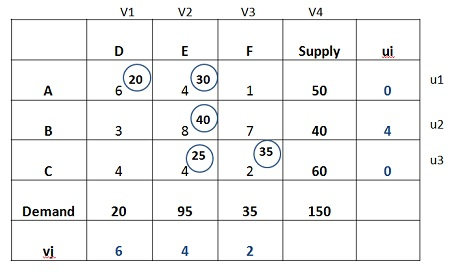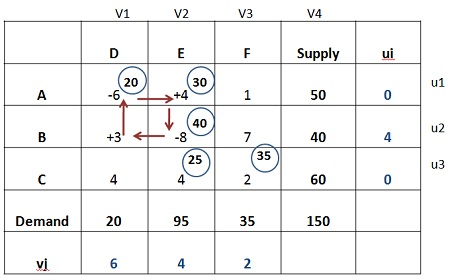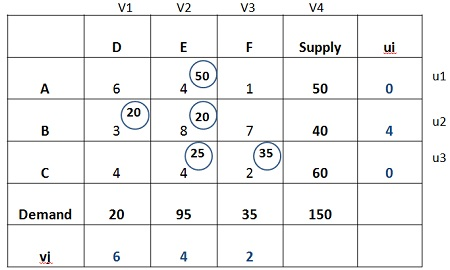# Modified Distribution Method (MODI Mothed)

The Modified Distribution Method or MODI is an efficient method of checking the optimality of the initial feasible solution.

The concept of MODI can be further comprehended through an illustration given below:

1. Initial basic feasible solution is given below2. Now, calculate the values of ui and vj by using the equation
ui+vj = Cij
Substituting the value of u1 as 0

U1+V1 = C11, 0+V1 = 6 or V1 = 6
U1 +V2 = C12, 0+V2 = 4 or V2 = 4
U2+V2 = C22, U2+4 = 8 or U2 = 4
U3+ V2 = C32, U3+4 = 4 or U3 = 0
U3+V3 = C33, 0+V3 = 2 or V3 =23. Next step is to calculate the opportunity cost of the unoccupied cells (AF, BD, BF, CD) by using the following formula
Cij – (ui+Vi)

AF = C13 – (U1+V3),  1- (0+2) = -1 or 1
BD = C21 – (U2+v1),   3- (4+6) = -7 or 7
BF = C23 – (U2+V3),   7- (4+2) = 1 or -1
CD = C31- (U3+V1),    4- (0+6) = -2 or 2

4. Choose the largest positive opportunity cost, which is 7 and draw a closed path, as shown in the matrix below. Start from the unoccupied cell and assign “+” or “–“sign alternatively. Therefore, The most favored cell is BD, assign as many units as possible.5. The matrix below shows the maximum allocation to the cell BD, and that number of units are added to the cell with a positive sign and subtracted from the cell with a negative sign.6. Again, repeat the steps from 1 to 4 i.e. find out the opportunity costs for each unoccupied cell and assign the maximum possible units to the cell having the largest opportunity cost. This process will go on until the optimum solution is reached.

The Modified distribution method is an improvement over the stepping stone method since; it can be applied more efficiently when a large number of sources and destinations are involved, which becomes quite difficult or tedious in case of stepping stone method.

Modified distribution method reduces the number of steps involved in the evaluation of empty cells, thereby minimizes the complexity and gives a straightforward computational scheme through which the opportunity cost of each empty cell can be determined.

## 3 thoughts on “Modified Distribution Method (MODI Mothed)”

error: Content is protected !!